# Data Visualization With R - Introduction

### Introduction

This is the first post of the series Data Visualization With R. The objective of the series is to provide a gentle introduction to working with base graphics in R. We will come up with a similar series using ggplot2 shortly.

• what is data visualization
• why visualize data
• understand R graphics system
• graphics
• ggplot2
• lattice
• build some simple plots

### Libraries, Code & Data

All the data sets used in this post can be found here and code can be downloaded from here.

### What is data visualization?

In simple words, data visualization is the representation of data in graphical format.data-viz

### Why visualize data?

• Explore: Visualization helps in exploring and explaining patterns and trends
• Detect: Patterns or anomalies in data can be detected by looking at graphs
• Make sense: Possible to make sense of large amount of data efficiently and in time
• Communicate: Easy to communicate and share the insights from data

### R Graphics System

• graphics
• ggplot2
• lattice

#### Graphics

• It is part of base R and is the fundamental package for visualizing data.
• It has a lot of good features and we can create all the basic plots using it.

#### ggplot2

ggplot2, created by Hadley Wickham, is based on the Grammar of Graphics written by Leland Wilkinson. It has a structured approach to data visualization and builds upon the features available in the Graphics and Lattice packages.

#### Lattice

The lattice package is inspired by Trellis Graphics and created by Deepayan Sarkar. It is a very powerful data visualization system with an emphasis on multivariate data.

### Getting Help

Use the help() to learn more about plot() function and mtcars data set.

help(plot)
help(mtcars)

### mtcars

head(mtcars)
##                    mpg cyl disp  hp drat    wt  qsec vs am gear carb
## Mazda RX4         21.0   6  160 110 3.90 2.620 16.46  0  1    4    4
## Mazda RX4 Wag     21.0   6  160 110 3.90 2.875 17.02  0  1    4    4
## Datsun 710        22.8   4  108  93 3.85 2.320 18.61  1  1    4    1
## Hornet 4 Drive    21.4   6  258 110 3.08 3.215 19.44  1  0    3    1
## Hornet Sportabout 18.7   8  360 175 3.15 3.440 17.02  0  0    3    2
## Valiant           18.1   6  225 105 2.76 3.460 20.22  1  0    3    1

### variable info

str(mtcars)
## 'data.frame':    32 obs. of  11 variables:
##  $mpg : num 21 21 22.8 21.4 18.7 18.1 14.3 24.4 22.8 19.2 ... ##$ cyl : num  6 6 4 6 8 6 8 4 4 6 ...
##  $disp: num 160 160 108 258 360 ... ##$ hp  : num  110 110 93 110 175 105 245 62 95 123 ...
##  $drat: num 3.9 3.9 3.85 3.08 3.15 2.76 3.21 3.69 3.92 3.92 ... ##$ wt  : num  2.62 2.88 2.32 3.21 3.44 ...
##  $qsec: num 16.5 17 18.6 19.4 17 ... ##$ vs  : num  0 0 1 1 0 1 0 1 1 1 ...
##  $am : num 1 1 1 0 0 0 0 0 0 0 ... ##$ gear: num  4 4 4 3 3 3 3 4 4 4 ...
##  $carb: num 4 4 1 1 2 1 4 2 2 4 ... ### plot() Now that we have some idea about the data set, let us explore the plot() function. We will use the following different data inputs and observe the kind of plots that are generated: • Case 1: 1 continuous variable • Case 2: 1 categorical variable • Case 3: 2 continuous variables • Case 4: 2 categorical variables • Case 5: 1 continuous and 1 categorical variable • Case 6: 1 categorical and 1 continuous variable ### One continuous variable plot(mtcars$mpg)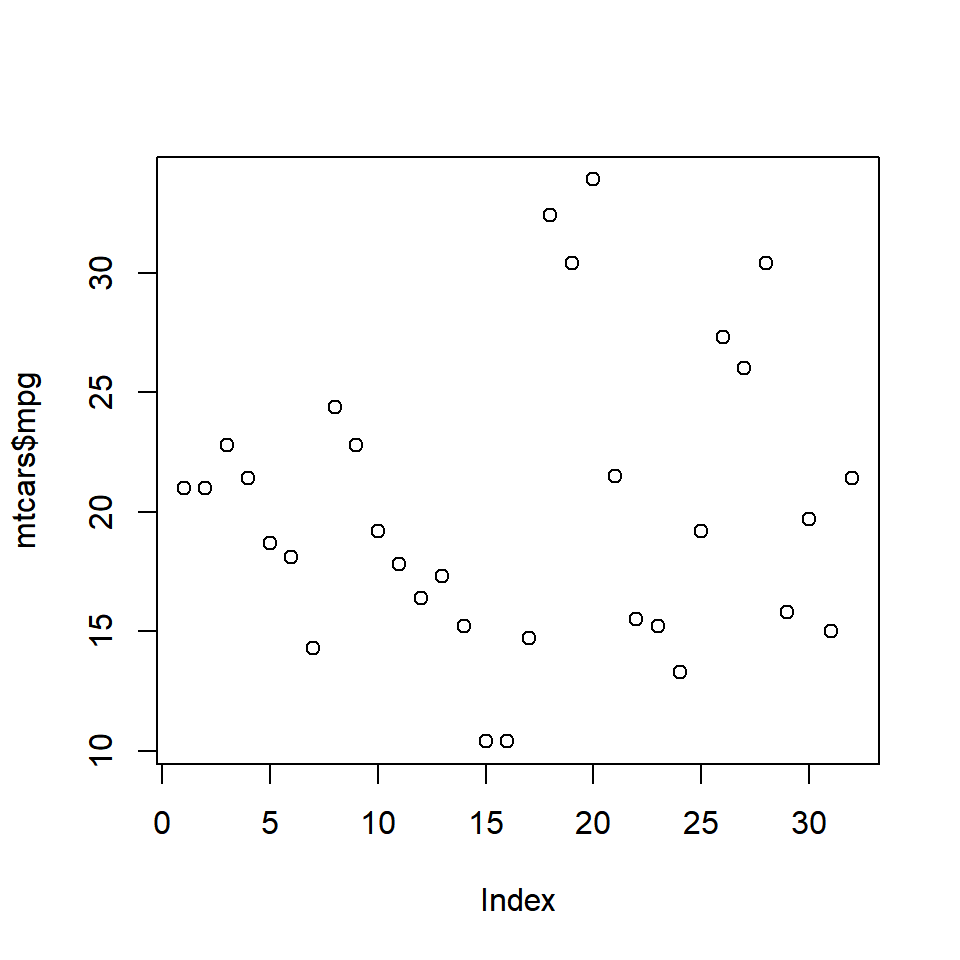plot(as.factor(mtcars$cyl))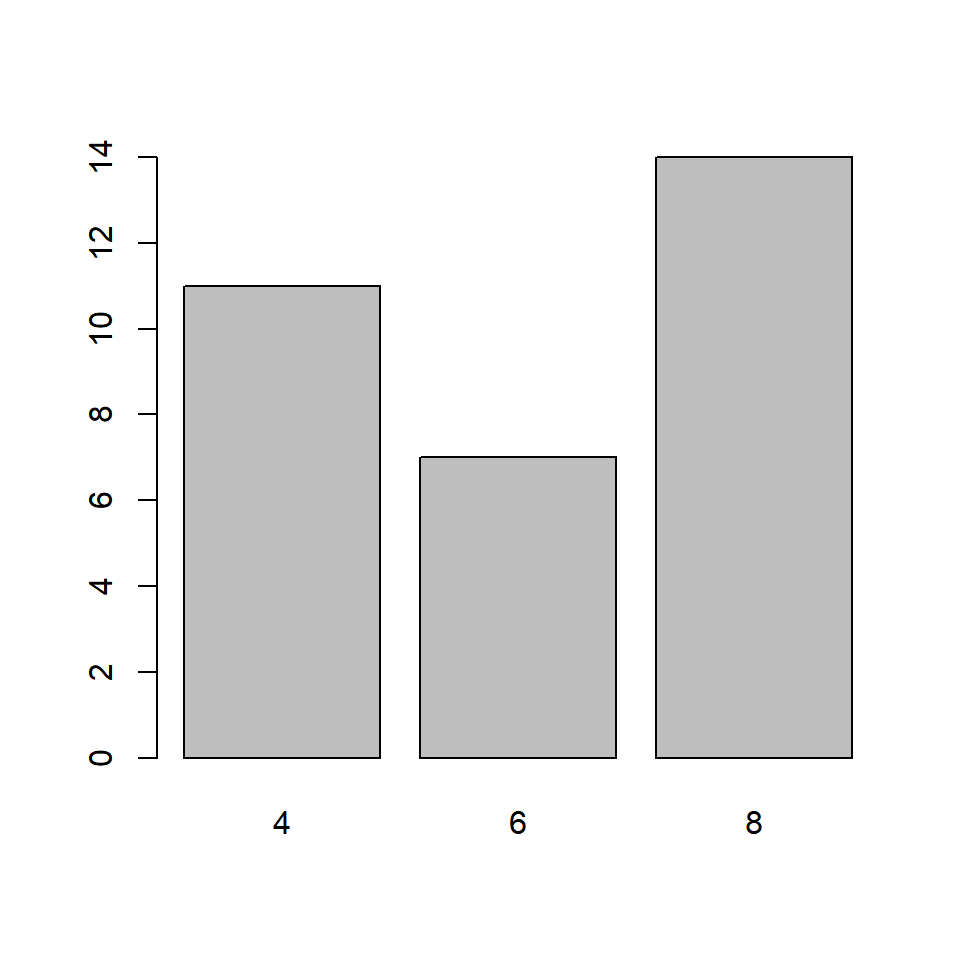### Two continuous variables plot(mtcars$disp, mtcars$mpg)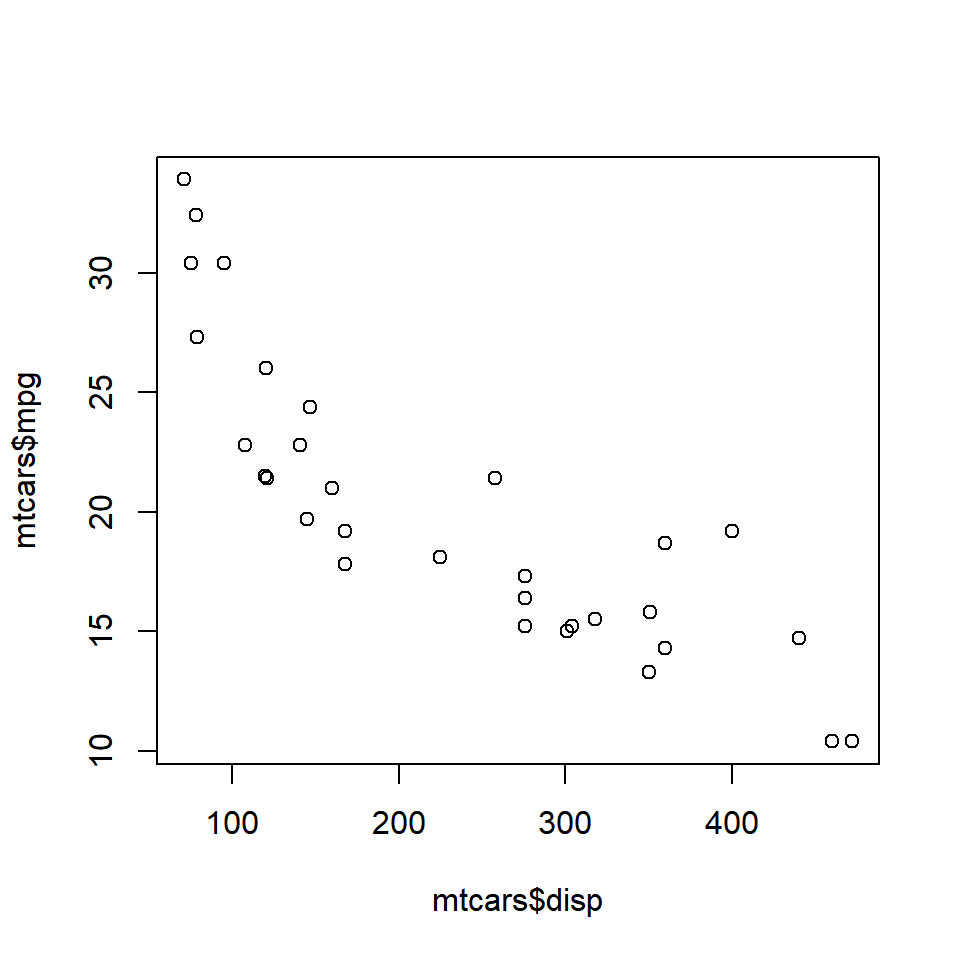### Two categorical variables plot(as.factor(mtcars$am), as.factor(mtcars$cyl))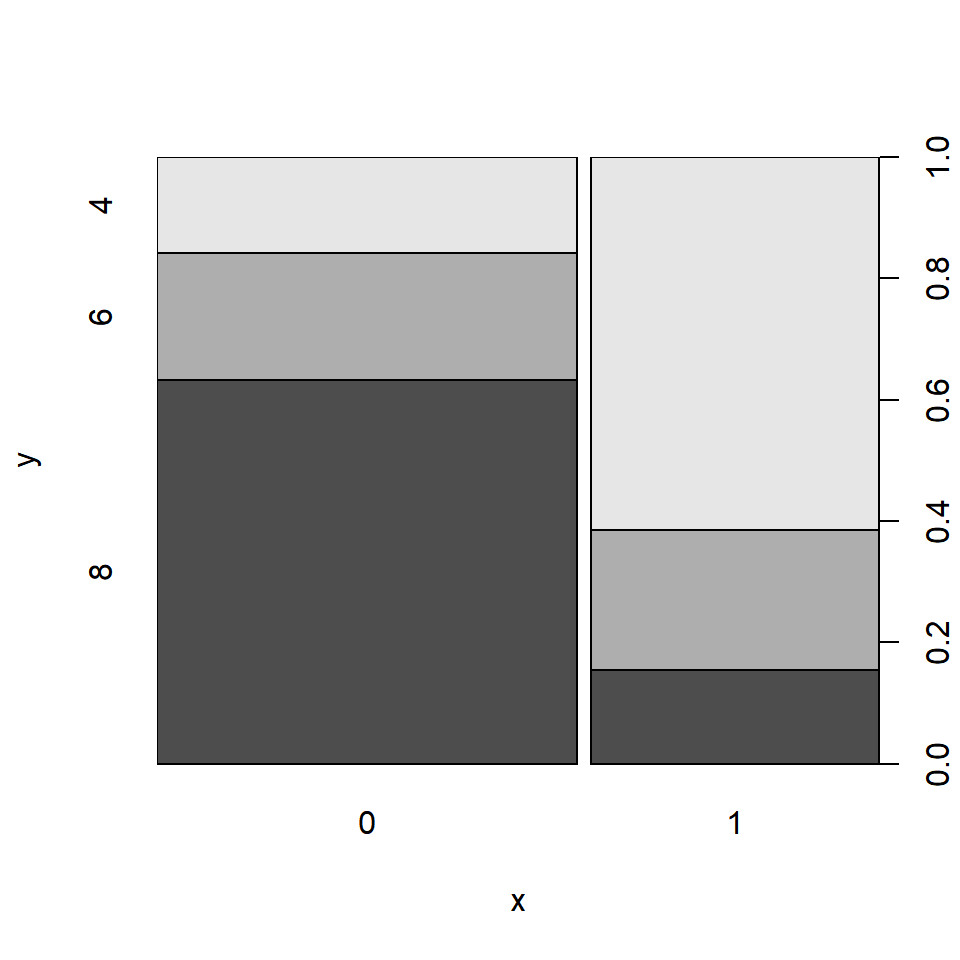### Continuous/Categorical variable plot(mtcars$mpg, mtcars$cyl)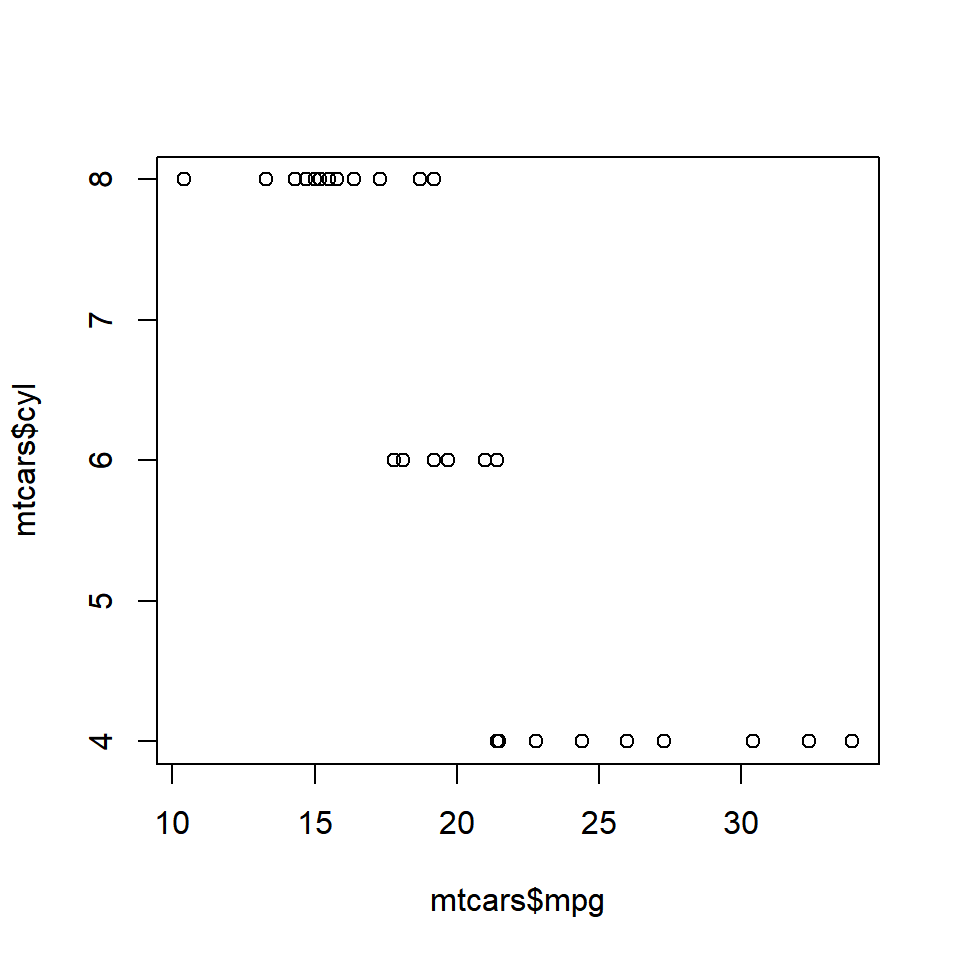### Categorical/Continuous variable plot(as.factor(mtcars$cyl), mtcars\$mpg)### Summary

In this first post, we have explored the plot() function to understand the different types of plots it can create based on the input types. Before we begin to build different plots such as bar plots, box plots, scatter plots or line plots, we will quickly learn how to add title and labels to a plot.